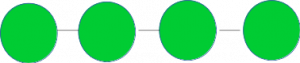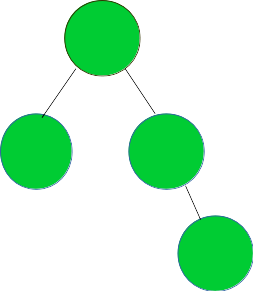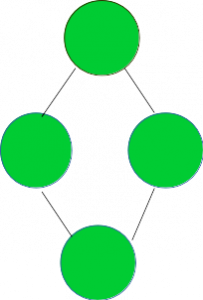# Check if the given graph represents a Bus Topology

Given a graph G, check if it represents a Bus Topology.

A Bus Topology is the one shown in the image below:Examples:

```Input:Output:  YES

Input:Output:  NO
```

## Recommended: Please try your approach on {IDE} first, before moving on to the solution.

A graph of V vertices represents a bus topology if it satisfies the following two conditions:

1. Each node except the stating end ending ones have degree 2 while the starting and ending have degree 1.
2. No of edges = No of Vertices – 1.

The idea is to traverse the graph and check if it satisfies the above two conditions. If yes, then it represents a Bus Topology.

Below is the implementation of the above approach:

## CPP

 `// CPP program to check if the given graph ` `// represents a bus topology ` `#include ` `using` `namespace` `std; ` ` `  `// A utility function to add an edge in an ` `// undirected graph. ` `void` `addEdge(vector<``int``> adj[], ``int` `u, ``int` `v) ` `{ ` `    ``adj[u].push_back(v); ` `    ``adj[v].push_back(u); ` `} ` ` `  `// A utility function to print the adjacency list ` `// representation of graph ` `void` `printGraph(vector<``int``> adj[], ``int` `V) ` `{ ` `    ``for` `(``int` `v = 0; v < V; ++v) { ` `        ``cout << ``"\n Adjacency list of vertex "` `             ``<< v << ``"\n head "``; ` `        ``for` `(``auto` `x : adj[v]) ` `            ``cout << ``"-> "` `<< x; ` `        ``printf``(``"\n"``); ` `    ``} ` `} ` ` `  `/* Function to return true if the graph represented ` `   ``by the adjacency list represents a bus topology ` `   ``else return false */` `bool` `checkBusTopologyUtil(vector<``int``> adj[], ``int` `V, ``int` `E) ` `{ ` ` `  `    ``// Number of edges should be equal ` `    ``// to (Number of vertices - 1) ` `    ``if` `(E != (V - 1)) ` `        ``return` `false``; ` ` `  `    ``// a single node is termed as a bus topology ` `    ``if` `(V == 1) ` `        ``return` `true``; ` ` `  `    ``int``* vertexDegree = ``new` `int``[V + 1]; ` `    ``memset``(vertexDegree, 0, ``sizeof` `vertexDegree); ` ` `  `    ``// calculate the degree of each vertex ` `    ``for` `(``int` `i = 1; i <= V; i++) { ` `        ``for` `(``auto` `v : adj[i]) { ` `            ``vertexDegree[v]++; ` `        ``} ` `    ``} ` ` `  `    ``// countDegree2 - number of vertices with degree 2 ` `    ``// countDegree1 - number of vertices with degree 1 ` `    ``int` `countDegree2 = 0, countDegree1 = 0; ` `    ``for` `(``int` `i = 1; i <= V; i++) { ` `        ``if` `(vertexDegree[i] == 2) { ` `            ``countDegree2++; ` `        ``} ` `        ``else` `if` `(vertexDegree[i] == 1) { ` `            ``countDegree1++; ` `        ``} ` `        ``else` `{ ` `            ``// if any node has degree other ` `            ``// than 1 or 2, it is ` `            ``// NOT a bus topology ` `            ``return` `false``; ` `        ``} ` `    ``} ` ` `  `    ``// if both necessary conditions as discussed, ` `    ``// satisfy return true ` `    ``if` `(countDegree1 == 2 && countDegree2 == (V - 2)) { ` `        ``return` `true``; ` `    ``} ` `    ``return` `false``; ` `} ` ` `  `// Function to check if the graph represents a bus topology ` `void` `checkBusTopology(vector<``int``> adj[], ``int` `V, ``int` `E) ` `{ ` `    ``bool` `isBus = checkBusTopologyUtil(adj, V, E); ` `    ``if` `(isBus) { ` `        ``cout << ``"YES"` `<< endl; ` `    ``} ` `    ``else` `{ ` `        ``cout << ``"NO"` `<< endl; ` `    ``} ` `} ` ` `  `// Driver code ` `int` `main() ` `{ ` `    ``// Graph 1 ` `    ``int` `V = 5, E = 4; ` `    ``vector<``int``> adj1[V + 1]; ` `    ``addEdge(adj1, 1, 2); ` `    ``addEdge(adj1, 1, 3); ` `    ``addEdge(adj1, 3, 4); ` `    ``addEdge(adj1, 4, 5); ` `    ``checkBusTopology(adj1, V, E); ` ` `  `    ``// Graph 2 ` `    ``V = 4, E = 4; ` `    ``vector<``int``> adj2[V + 1]; ` `    ``addEdge(adj2, 1, 2); ` `    ``addEdge(adj2, 1, 3); ` `    ``addEdge(adj2, 3, 4); ` `    ``addEdge(adj2, 4, 2); ` `    ``checkBusTopology(adj2, V, E); ` ` `  `    ``return` `0; ` `} `

## Python3

 `# Python3 program to check if the given graph ` `# represents a bus topology ` ` `  `# A utility function to add an edge in an ` `# undirected graph. ` `def` `addEdge(adj, u, v): ` `    ``adj[u].append(v) ` `    ``adj[v].append(u) ` ` `  `# A utility function to prthe adjacency list ` `# representation of graph ` `def` `printGraph(adj, V): ` ` `  `    ``for` `v ``in` `range``(V): ` `        ``print``(``"Adjacency list of vertex "``,v,``"\n head "``) ` `        ``for` `x ``in` `adj[v]: ` `            ``print``(``"-> "``,x,end``=``" "``) ` `        ``printf() ` ` `  `# /* Function to return true if the graph represented ` `# by the adjacency list represents a bus topology ` `# else return false */ ` `def` `checkBusTopologyUtil(adj, V, E): ` ` `  `    ``# Number of edges should be equal ` `    ``# to (Number of vertices - 1) ` `    ``if` `(E !``=` `(V ``-` `1``)): ` `        ``return` `False` ` `  `    ``# a single node is termed as a bus topology ` `    ``if` `(V ``=``=` `1``): ` `        ``return` `True` ` `  `    ``vertexDegree ``=` `[``0``]``*``(V ``+` `1``) ` ` `  `    ``# calculate the degree of each vertex ` `    ``for` `i ``in` `range``(V ``+` `1``): ` `        ``for` `v ``in` `adj[i]: ` `            ``vertexDegree[v] ``+``=` `1` ` `  `    ``# countDegree2 - number of vertices with degree 2 ` `    ``# countDegree1 - number of vertices with degree 1 ` `    ``countDegree2,countDegree1 ``=` `0``,``0` `    ``for` `i ``in` `range``(``1``, V ``+` `1``): ` `        ``if` `(vertexDegree[i] ``=``=` `2``): ` `            ``countDegree2 ``+``=` `1` ` `  `        ``elif` `(vertexDegree[i] ``=``=` `1``): ` `            ``countDegree1 ``+``=` `1` ` `  `        ``else``: ` `            ``# if any node has degree other ` `            ``# than 1 or 2, it is ` `            ``# NOT a bus topology ` `            ``return` `False` ` `  `    ``# if both necessary conditions as discussed, ` `    ``# satisfy return true ` `    ``if` `(countDegree1 ``=``=` `2` `and` `countDegree2 ``=``=` `(V ``-` `2``)): ` `        ``return` `True` ` `  `    ``return` `False` ` `  `# Function to check if the graph represents a bus topology ` `def` `checkBusTopology(adj, V, E): ` ` `  `    ``isBus ``=` `checkBusTopologyUtil(adj, V, E) ` `    ``if` `(isBus): ` `        ``print``(``"YES"``) ` ` `  `    ``else``: ` `        ``print``(``"NO"` `) ` ` `  `# Driver code ` ` `  `# Graph 1 ` `V, E ``=` `5``, ``4` `adj1 ``=` `[[] ``for` `i ``in` `range``(V ``+` `1``)] ` `addEdge(adj1, ``1``, ``2``) ` `addEdge(adj1, ``1``, ``3``) ` `addEdge(adj1, ``3``, ``4``) ` `addEdge(adj1, ``4``, ``5``) ` `checkBusTopology(adj1, V, E) ` ` `  `# Graph 2 ` `V, E ``=` `4``, ``4` `adj2 ``=` `[[] ``for` `i ``in` `range``(V ``+` `1``)] ` `addEdge(adj2, ``1``, ``2``) ` `addEdge(adj2, ``1``, ``3``) ` `addEdge(adj2, ``3``, ``4``) ` `addEdge(adj2, ``4``, ``2``) ` `checkBusTopology(adj2, V, E) ` ` `  `# This code is contributed by mohit kumar 29 `

Output:

```YES
NO
```

Time Complexity : O(E), where E is the number of Edges in the graph.My Personal Notes arrow_drop_upCheck out this Author's contributed articles.

If you like GeeksforGeeks and would like to contribute, you can also write an article using contribute.geeksforgeeks.org or mail your article to contribute@geeksforgeeks.org. See your article appearing on the GeeksforGeeks main page and help other Geeks.

Please Improve this article if you find anything incorrect by clicking on the "Improve Article" button below.

Improved By : mohit kumar 29